# GeoGebra软件在高等数学教学中的应用On Application of GeoGebra Software in Higher Mathematics Teaching

DOI: 10.12677/CES.2021.91026, PDF, HTML, XML, 下载: 54  浏览: 111

Abstract: GeoGebra, a software applied in advanced mathematics teaching, which transforms the abstract theoretical knowledge into a dynamic demonstration of visual image, can bring vitality into teaching and improve the teaching quality. Based on the volume of rotational solid as an example, this paper discusses the application of GeoGebra in higher mathematics teaching.

1. 引言

GeoGebra是数学教授Markus Hohenwarter于2002年创建的动态教学软件，并通过开源的方式不断地更新、完善和推广。GeoGebra功能强大、操作简单、资源丰富，能够进行动态交互，可脱机、可在线，能跨平台使用，且占用空间极小，在欧美等国家已获数十项相关领域的大奖 。GeoGebra在动态显示、直观性、精确性方面表现都非常优秀。GeoGebra 5.0 beta版本新增加的3D功能，可以制作空间立体图形，而且支持几何变换，可以多角度观察立体。将其与高等数学教学相结合，可收到事半功倍的效果。

2. 基于“切片法”，利用GeoGebra动态演示旋转体的形成过程

2.1. 实例1的计算方法

x轴旋转， ${V}_{x}={\int }_{0}^{4}\pi {y}^{2}\left(x\right)\text{d}x={\int }_{0}^{4}\pi \left(4-x\right)\text{d}x=8\pi$ (1)

y轴旋转， ${V}_{y}={\int }_{0}^{2}\pi {x}^{2}\left(y\right)\text{d}y={\int }_{0}^{2}\pi {\left(4-{y}^{2}\right)}^{2}\text{d}y=\frac{256}{15}\pi$ (2)

2.2. 关于实例1的GeoGebra软件操作步骤

1) 分别打开绘图区、3D绘图区、代数区。

2) 在绘图区建立滑动条t，类型为角度，范围0度到360度；建立滑动条m，n，类型为数值，范围0到1，增量为0.01。

3) 指令栏分别输入：

f(x) = 如果(0 ≤ x ≤ 4, sqrt(4 - x))，A = (0, 2)，B = (4, 0)，O = (0, 0)，a = 线段(A, O)，b = 线段(O, B)，

c: 0 ≤ x ≤ 4 Ù 0 ≤ y ≤ sqrt(4 - x)。

4) 指令栏分别输入：

d = 曲面(a, t, x轴)，e = 曲面(f, t, x轴)，g = 旋转(f, t, x轴)。

5) 指令兰分别输入：

C = 描点(b, m)，D = (x(C), sqrt(4 - x(C)))，E = 描点(f, n)，F = (x(E), 0)，h = 线段(C, D)，i = 线段(E, F)，

f_1 = 如果(x(F) ≤ x ≤ x(C), sqrt(4 - x))，j:x(E) ≤ x ≤ x(D) Ù 0 ≤ y ≤ sqrt(4 - x)。

6) 指令栏分别输入：

k = 曲面(f_1, t, x轴)，p = 曲面(线段(F, E), t, x轴)，q = 曲面(线段(D, C), t, x轴)。

7) 指令栏分别输入：

r = 曲面(b, t, y轴)，s = 曲面(f, t, y轴)。

8) 在绘图区建立“复选框”，在下拉列表中选取“曲面e”，标题改为“外部曲面”。

2.3. 操作说明

2.4. 切片法的微积分思想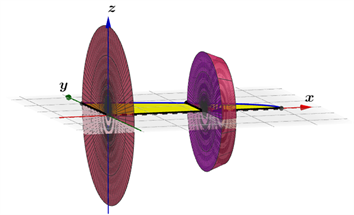Figure 1. In Example 1, the solid formed by rotation of the element graph region about the x axis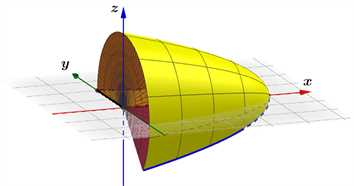Figure 2. In Example 1, the process by which the enclosed plane region rotates about the x axis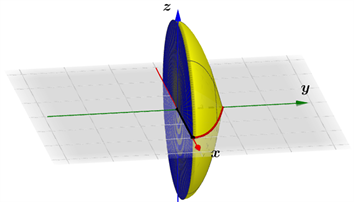Figure 3. In Example 1, a solid formed by rotation of the enclosed plane region about the y axis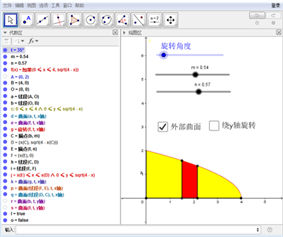Figure 4. Algebra area and plot area of Example 1

3. 基于“扣洞法”，利用GeoGebra动态演示旋转体的形成过程

3.1. 实例2的计算方法

3.2. 关于实例2的GeoGebra软件操作步骤

1) 分别打开绘图区、3D绘图区、代数区。

2) 在绘图区建立滑动条t，类型为角度，范围0度到360度。

3) 指令栏分别输入：

f(x) = 如果(2 ≤ x ≤ 6, −0.5(x − 4)2 + 2)，g(x) = 如果(2 ≤ x ≤ 4, −0.5(x − 4)2 + 2)，

h(x) = 如果(4 ≤ x ≤ 6, −0.5(x − 4)2 + 2)。

4) 指令栏分别输入：

A = (2, 0)，B = (6, 0)，C = (4, 2)，D = (0, 2)，O = (0, 0)，

a = 线段(A, O)，b = 线段(B, O)，c = 线段(C, D)，d = 线段(O, D)，e = 线段(B, A)，

i: 2 ≤ x ≤ 6 Ù 0 ≤ y ≤−0.5(x − 4)2 + 2。

5) 指令栏分别输入：

j = 曲面(g, t, y轴)，k = 曲面(h, t, y轴)，l = 曲面(e, t, y轴)，m = 曲面(c, t, y轴)，n = 曲面(a, t, y轴)。

6) 指令栏分别输入：

p = 曲面(ucos(t), sf(u), -usin(t), u, 2, 4, s, 0, 1)，q = 曲面(ucos(t), sf(u), -usin(t), u, 4, 6, s, 0, 1)。

7) 在绘图区建立“复选框”，在下拉列表中选取“曲面k”，标题改为“外部曲面”在绘图区建立“复选框”，在下拉列表中选取“曲面m”，标题改为“内部立体”。

3.3. 操作说明

3.4. 旋转体的体积公式(3)的数学本质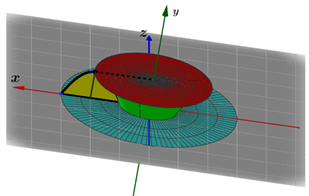Figure 5. The internal structure of Formula (3)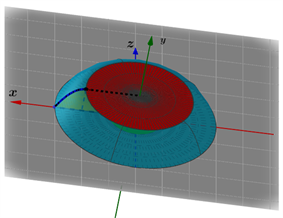Figure 6. The overall structure of Formula (3)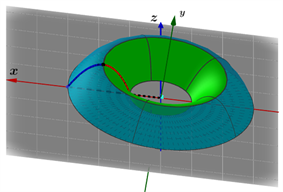Figure 7. In Formula (3), a solid formed by rotation of the enclosed plane region about the y axis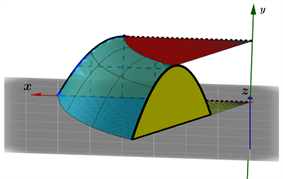Figure 8. In Formula (3), the process by which the enclosed plane region rotates about the y axis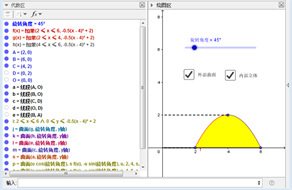Figure 9. Algebraic area and plot area of Formula (3)

4. 基于“套筒法”，利用GeoGebra动态演示旋转体的形成过程

4.1. 关于实例2的GeoGebra软件操作步骤

1) 分别打开绘图区、3D绘图区、代数区。

2) 在绘图区建立滑动条t，类型为角度，范围0度到360度；滑动条m，n，类型为数值，范围0到1，增量为0.01。

3) 指令栏分别输入：

f(x) = 如果(2 ≤ x ≤ 6, −0.5(x − 4)2 + 2)，A = (2, 0)，B = (6, 0)，a = 线段(A, B)，b = 线段(A, B)，

s: 2 ≤ x ≤ 6 Ù 0 ≤ y ≤ −0.5(x − 4)2 + 2。

4) 指令栏分别输入：

c = 曲面(f, t, y轴)，d = 曲面(a, t, y轴)。

5) 指令栏分别输入：

C = 描点(a, m)，D = 描点(b, n)，E = (x(C), f(x(C)))，F = (x(D), f(x(D)))，e = 线段(C, E)，g = 线段(D, F)，h = 线段(C, D)，i = 如果(x(C) ≤ x ≤ x(D), −0.5(x − 4)2 + 2), j:x(C) ≤ x ≤ x(D) Ù 0 ≤ y ≤ −0.5(x − 4)2 + 2。

6) 指令栏分别输入：

k = 曲面(e, t, y轴)，p = 曲面(g, t, y轴)，q = 曲面(h, t, y轴)，r = 曲面(i, t, y轴)。

7) 指令栏分别输入：

v = 曲面(ucos(t), sf(u), -usin(t), u, 2, 6, s, 0, 1)，w = 曲面(ucos(t), sf(u), -usin(t), u, x(C), x(D), s, 0, 1)。

4.2. 操作说明

4.3. 套筒法的微积分思想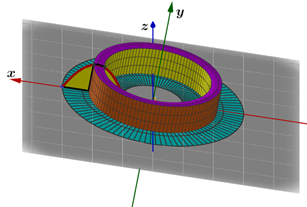Figure 10. In Formula (4), the solid formed by rotation of the element graph region about the y axisFigure 11. In Formula (4), the process by which the enclosed plane region rotates about the y axisFigure 12. Algebraic area and plot area of Formula (4)

5. 结束语

GeoGebra动态数学软件将代数窗口与图形绘制窗口完美结合，在高等数学课程的教学过程中表现卓越，大大提高了学生的学习兴趣与热情，丰富了课堂内容。利用GeoGebra数学软件进行辅助教学，将高等数学概念的形成过程进行思维可视化，使学生透过抽象的公式，看到它所代表的图形以及深刻的微积分思想，不但对数学表达式理解更透测，而且能够感受到数学语言的魅力。教师在高等数学课程的教学过程中利用GeoGebra软件设计教学方案，创建用作演示的交互式应用程序，将传统的教学观念与模式进行改革创新，进而提高课程的教学质量。

  王贵军. GeoGebra与数学实验[M]. 北京: 清华大学出版社, 2017.  同济大学数学系. 高等数学上册[M]. 第7版. 北京: 高等教育出版社, 2014.  闫永芳. GeoGebra软件在大学文科数学教学中的应用[J]. 山东商业职业技术学院学报, 2018, 18(5): 62-64+71.  马玉青. GeoGebra软件在高等数学课程教学上的运用[J]. 现代农村科技, 2018(10): 87.  牛海军. 数学软件GeoGebra在高等数学教学中的应用[J]. 牡丹江教育学院学报. 2016(1): 63-64.